NCERT Questions

Class 9
Chapter 11 Class 9 - Work and Energy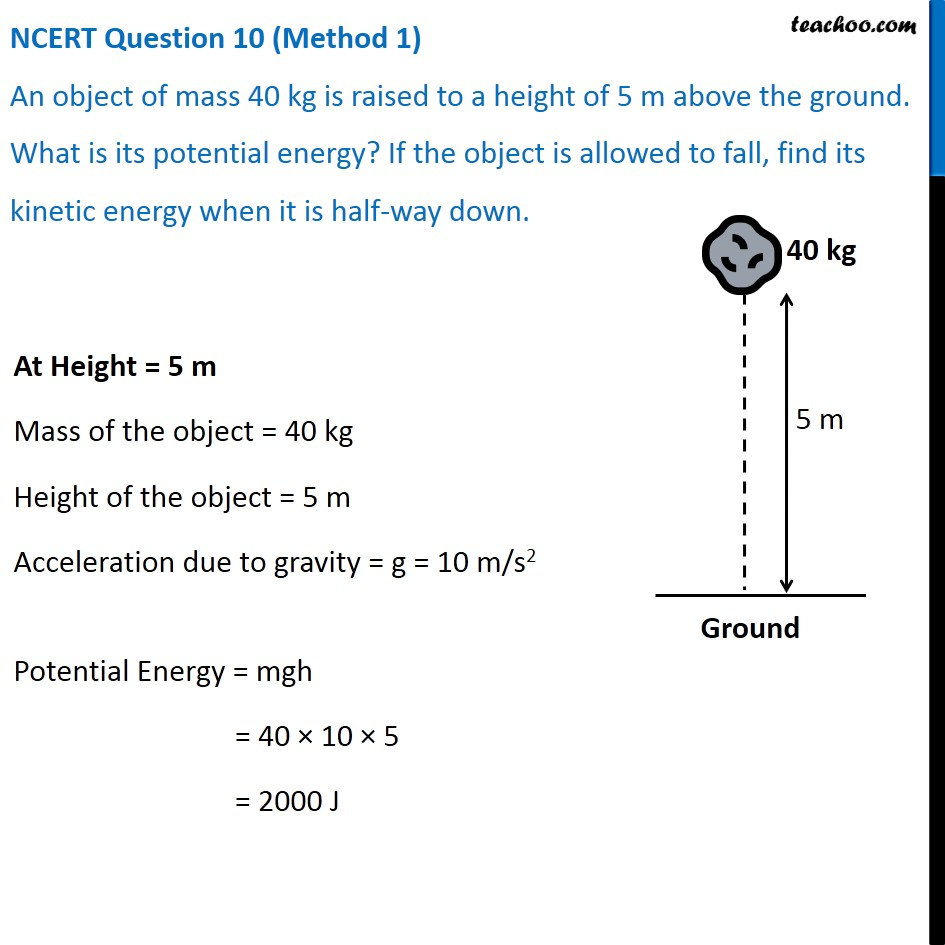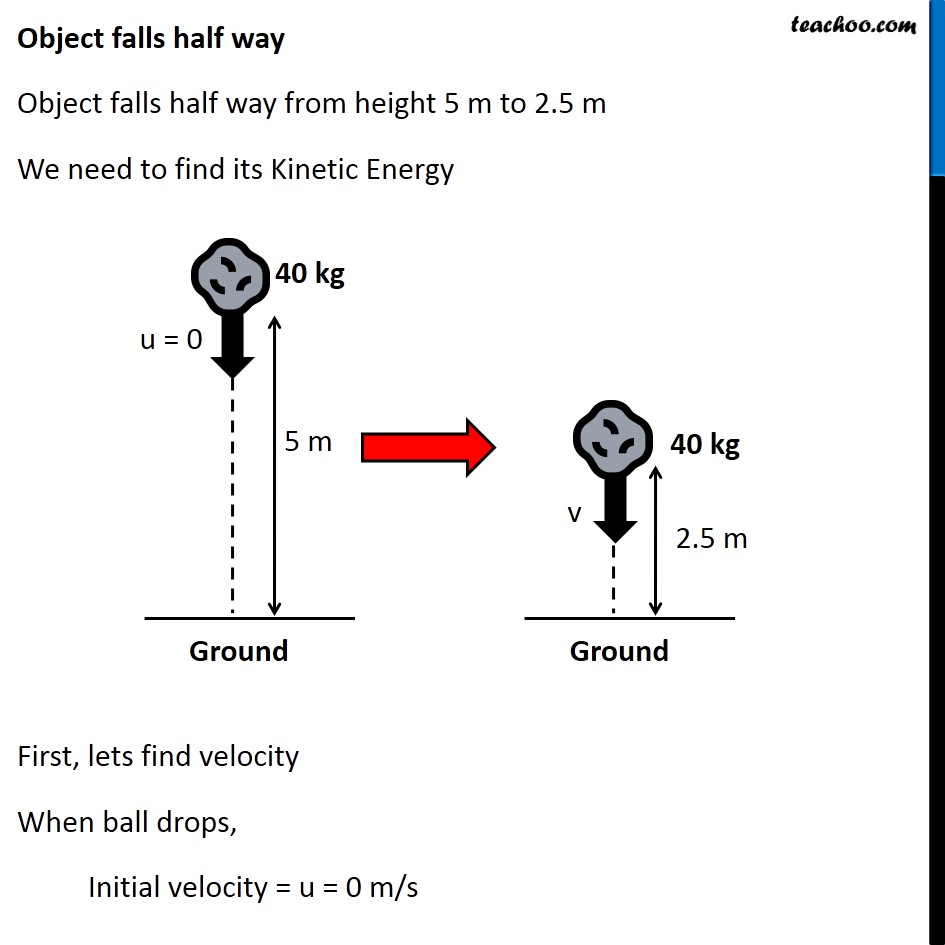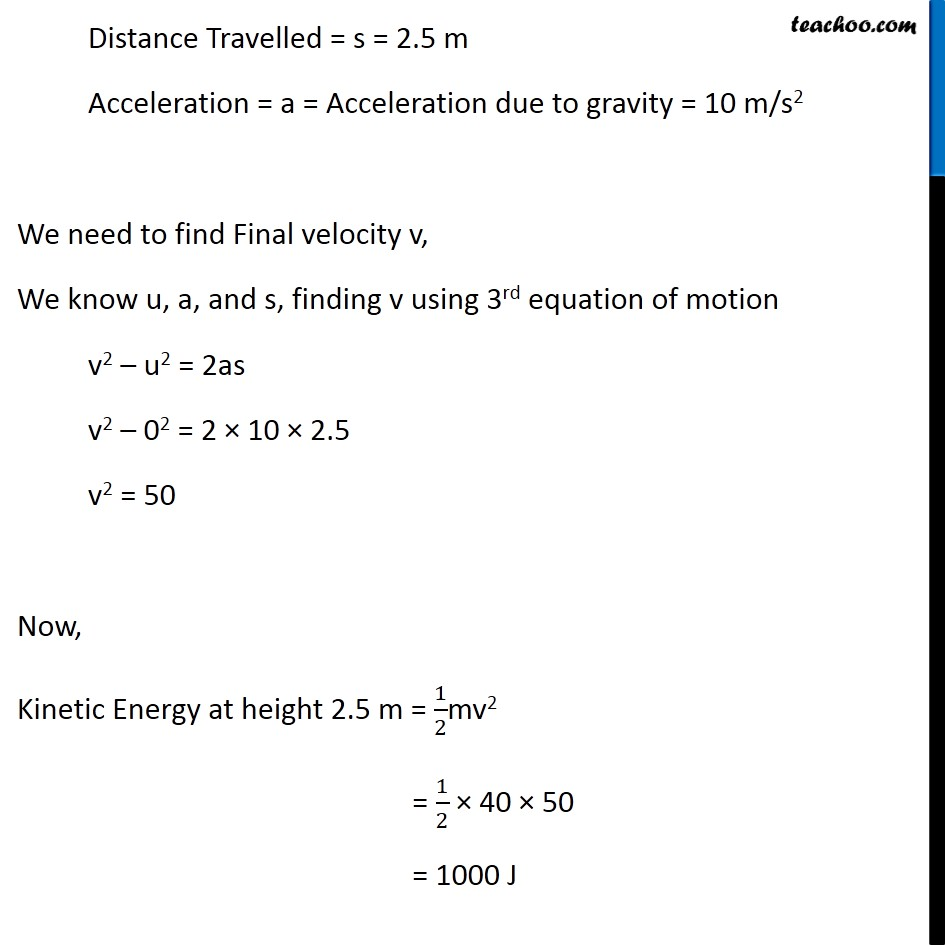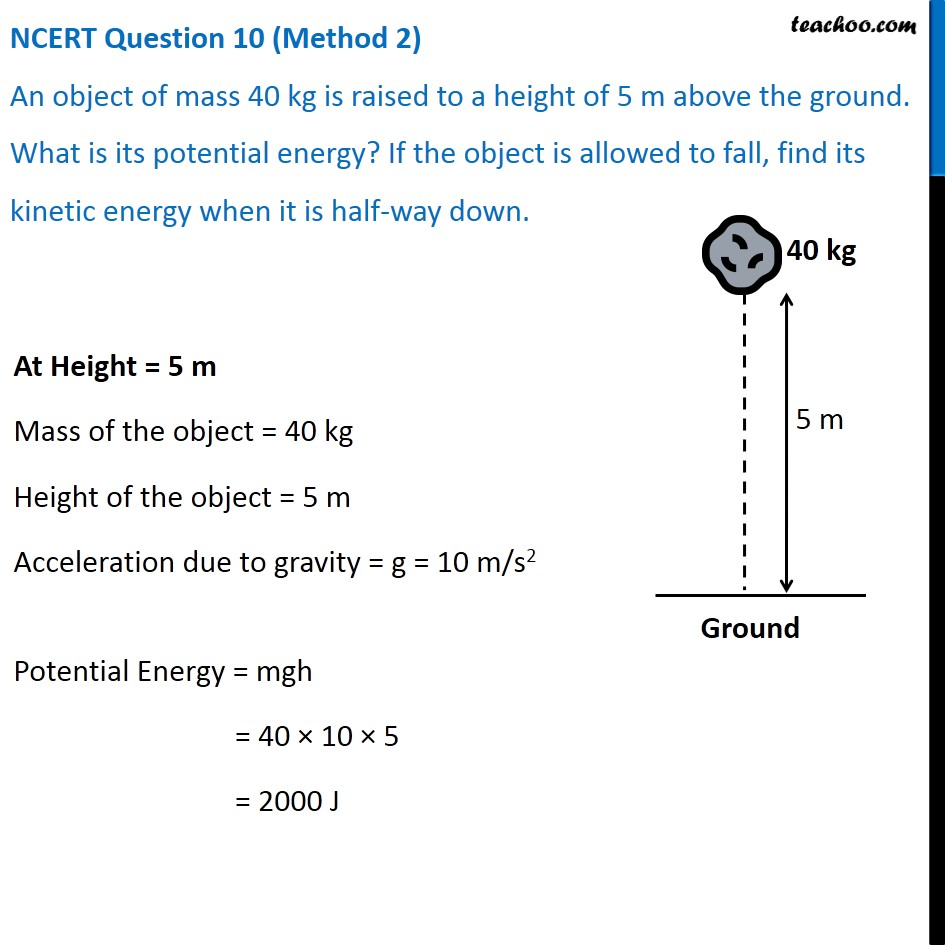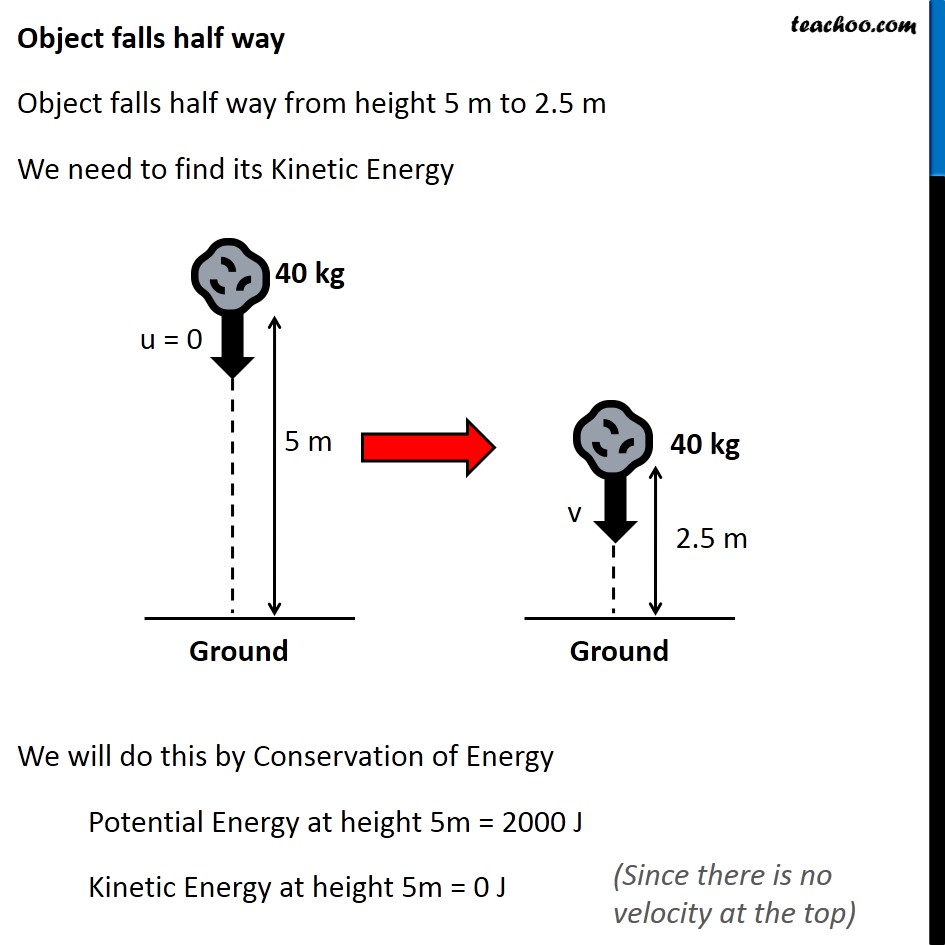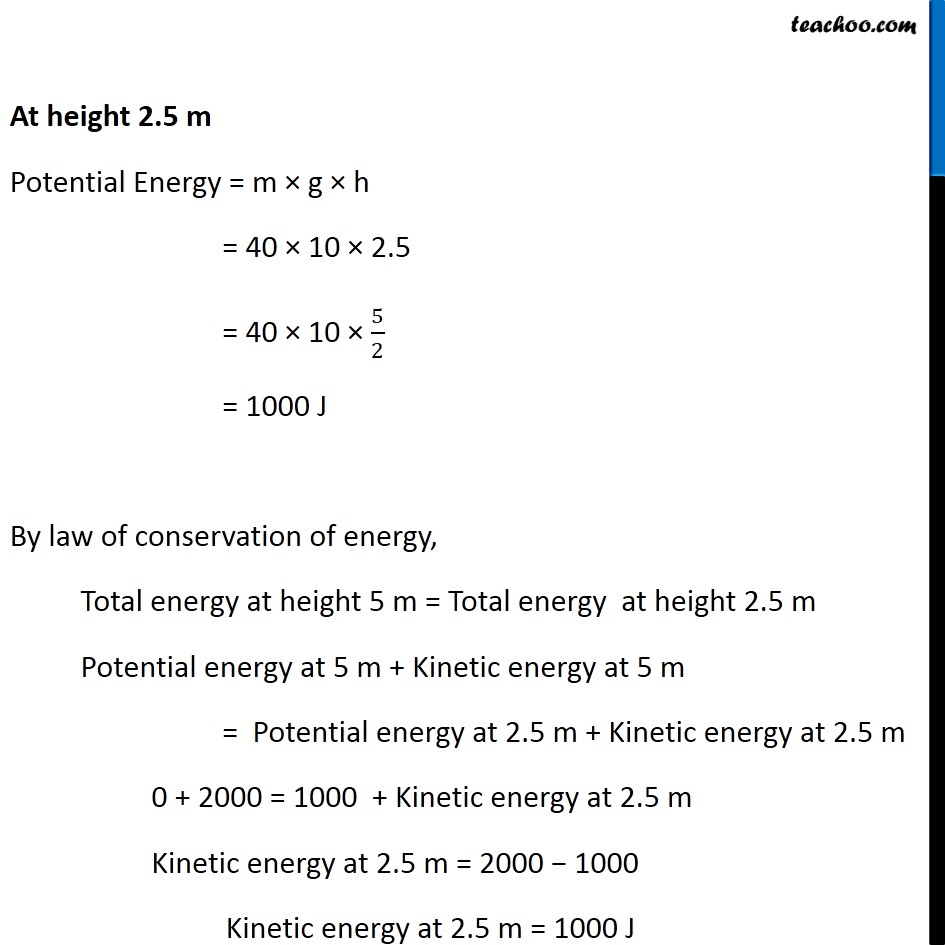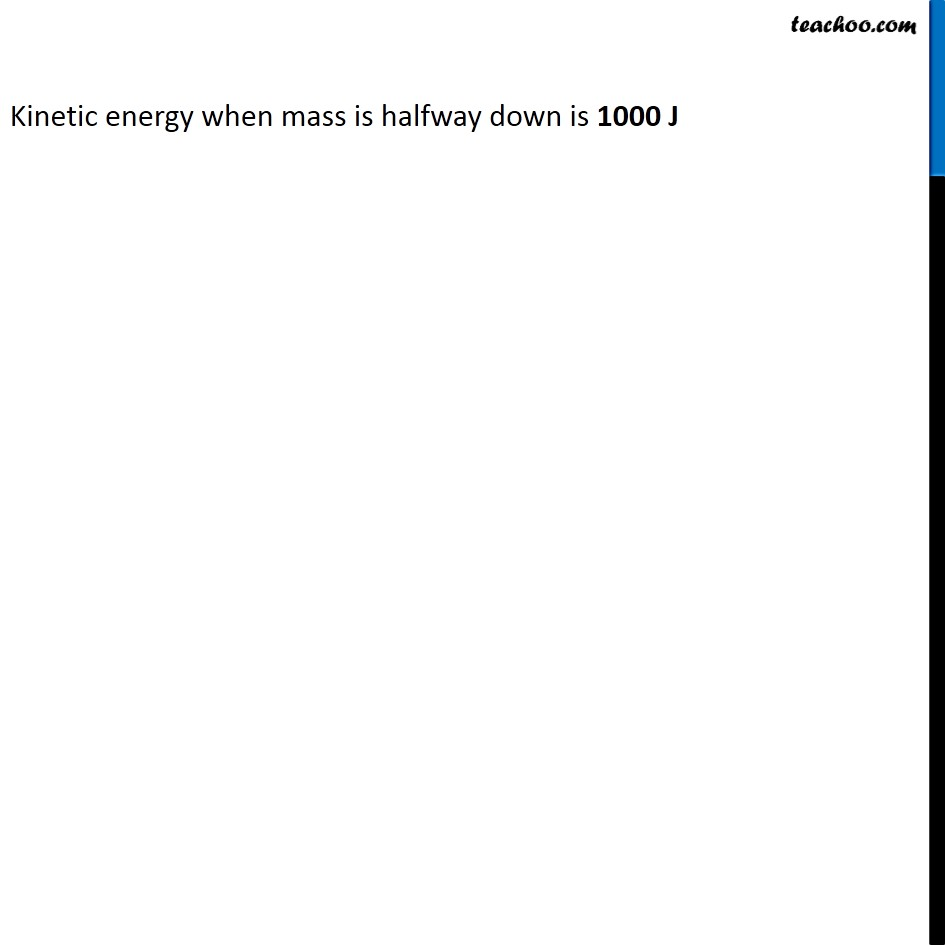Learn in your speed, with individual attention - Teachoo Maths 1-on-1 Class

### Transcript

NCERT Question 10 (Method 1) An object of mass 40 kg is raised to a height of 5 m above the ground. What is its potential energy? If the object is allowed to fall, find its kinetic energy when it is half-way down. At Height = 5 m Mass of the object = 40 kg Height of the object = 5 m Acceleration due to gravity = g = 10 m/s2 Potential Energy = mgh = 40 × 10 × 5 = 2000 J Object falls half way Object falls half way from height 5 m to 2.5 m We need to find its Kinetic Energy First, lets find velocity When ball drops, Initial velocity = u = 0 m/s Distance Travelled = s = 2.5 m Acceleration = a = Acceleration due to gravity = 10 m/s2 We need to find Final velocity v, We know u, a, and s, finding v using 3rd equation of motion v2 – u2 = 2as v2 – 02 = 2 × 10 × 2.5 v2 = 50 Now, Kinetic Energy at height 2.5 m = 1/2mv2 = 1/2 × 40 × 50 = 1000 J NCERT Question 10 (Method 2) An object of mass 40 kg is raised to a height of 5 m above the ground. What is its potential energy? If the object is allowed to fall, find its kinetic energy when it is half-way down. At Height = 5 m Mass of the object = 40 kg Height of the object = 5 m Acceleration due to gravity = g = 10 m/s2 Potential Energy = mgh = 40 × 10 × 5 = 2000 J Object falls half way Object falls half way from height 5 m to 2.5 m We need to find its Kinetic Energy We will do this by Conservation of Energy Potential Energy at height 5m = 2000 J Kinetic Energy at height 5m = 0 J (Since there is no velocity at the top) At height 2.5 m Potential Energy = m × g × h = 40 × 10 × 2.5 = 40 × 10 × 5/2 = 1000 J By law of conservation of energy, Total energy at height 5 m = Total energy at height 2.5 m Potential energy at 5 m + Kinetic energy at 5 m = Potential energy at 2.5 m + Kinetic energy at 2.5 m 0 + 2000 = 1000 + Kinetic energy at 2.5 m Kinetic energy at 2.5 m = 2000 − 1000 Kinetic energy at 2.5 m = 1000 J Kinetic energy when mass is halfway down is 1000 J Kinetic energy when mass is halfway down is 1000 J